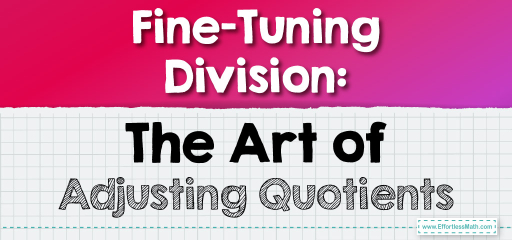# Fine-Tuning Division: The Art of Adjusting Quotients

In the world of division, sometimes our initial estimates or calculations might not be spot on. This is where the concept of adjusting quotients comes into play. Adjusting quotients is all about refining our initial estimates to get to the correct quotient. Let's delve into how and why we might need to adjust quotients.

When we divide, especially with larger numbers, we often start with an estimate. If our estimate is too high or too low, we adjust it to get the correct answer. This is particularly common when using methods like partial quotients.### Example 1:

Divide $$235$$ by $$7$$.

Initial Estimate:

You might start by thinking $$7$$ goes into $$235$$ about $$30$$ times because $$7 \times 30 = 210$$.

After subtracting $$210$$ from $$235$$, you have a remainder of $$25$$. Since $$7$$ can go into $$25$$ three more times, you adjust your quotient from $$30$$ to $$33$$.

The quotient is $$33$$ with a remainder of $$4$$.

The Absolute Best Book for 5th Grade Students

### Example 2:

Divide $$525$$ by $$23$$.

Initial Estimate:

You might start by thinking $$23$$ goes into $$525$$ about $$20$$ times because $$23 \times 20 = 460$$.

After subtracting $$460$$ from $$525$$, you have $$65$$ left. Since $$23$$ can go into $$65$$ two more times, you adjust your quotient from $$20$$ to $$22$$.

The quotient is $$22$$ with a remainder of $$19$$.

Adjusting quotients is a crucial skill in division, allowing for more accurate results. It emphasizes the importance of estimation, understanding of multiplication, and the iterative nature of refining answers. With practice, you’ll become adept at making these adjustments swiftly and accurately, ensuring your division results are always on point!

### Practice Questions:

1. Divide $$348$$ by $$12$$. Start with an estimate and adjust your quotient.

2. Divide $$640$$ by $$25$$. Begin with an estimate and refine your answer.

3. Divide $$299$$ by $$9$$. Estimate first, then adjust to get the correct quotient.

4. Divide $$444$$ by $$18$$. Start with an initial guess and adjust as needed.

5. Divide $$720$$ by $$29$$. Begin with an estimate and refine your quotient.

A Perfect Book for Grade 5 Math Word Problems!

1. Initial estimate: $$12 \times 25 = 300$$. Adjusted quotient: $$29$$ with a remainder of $$0$$.

2. Initial estimate: $$25 \times 24 = 600$$. Adjusted quotient: $$25$$ with a remainder of $$15$$.

3. Initial estimate: $$9 \times 30 = 270$$. Adjusted quotient: $$33$$ with a remainder of $$2$$.

4. Initial estimate: $$18 \times 24 = 432$$. Adjusted quotient: $$24$$ with a remainder of $$12$$.

5. Initial estimate: $$29 \times 24 = 696$$. Adjusted quotient: $$24$$ with a remainder of $$24$$.

The Best Math Books for Elementary Students

### What people say about "Fine-Tuning Division: The Art of Adjusting Quotients - Effortless Math: We Help Students Learn to LOVE Mathematics"?

No one replied yet.

X
51% OFF

Limited time only!

Save Over 51%

SAVE $15 It was$29.99 now it is \$14.99